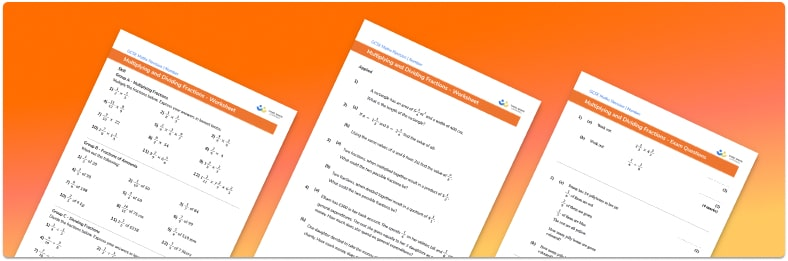# Multiplying And Dividing Fractions Worksheet• Section 1 of the Multiplying and Dividing Fractions worksheet contains 12 skills-based Multiplying and Dividing Fractions questions, in 3 groups to support differentiation
• Section 2 contains 4 applied Multiplying and Dividing Fractions questions with a mix of worded problems and deeper problem solving questions
• Section 3 contains 4 foundation and higher level GCSE exam style Multiplying and Dividing Fractions questions
• Answers and a mark scheme for all Multiplying and Dividing Fractions questions
• Follows variation theory with plenty of opportunities for students to work independently at their own level
• All questions created by fully qualified expert secondary maths teachers
• Suitable for GCSE maths revision for AQA, OCR and Edexcel exam boards

• This field is for validation purposes and should be left unchanged.

You can unsubscribe at any time (each email we send will contain an easy way to unsubscribe). To find out more about how we use your data, see our privacy policy.

### Multiplying and dividing fractions at a glance

Multiplication and division of fractions can be more straightforward than adding or subtracting fractions because a common denominator is not required. It is important however that students are confident with equivalent fractions and simplifying fractions.

To multiply a pair of fractions, simply multiply the numerators and multiply the denominators. The answer should always be written in its simplest form by cancelling common factors from the numerator and denominator.

To divide a pair of fractions, we find the reciprocal of the second fraction (essentially, flip the numerator and denominator). We then multiply the first fraction by the reciprocal of the second fraction, and finally cancel any common factors to write the fraction in its lowest terms.

Fraction multiplication and division can be extended to working with mixed numbers (sometimes called mixed fractions); a mixed number has both a whole number (integer) part and a simple fraction part. We can convert between mixed numbers and improper fractions in order to calculate with them.

Looking forward, students can then progress to additional number worksheets, for example aor aFor more teaching and learning support on Number , our GCSE maths lessons provide step by step support for all GCSE maths concepts.

## Do you have KS4 students who need more focused attention to succeed at GCSE?There will be students in your class who require individual attention to help them succeed in their maths GCSEs. In a class of 30, it’s not always easy to provide.

Help your students feel confident with exam-style questions and the strategies they’ll need to answer them correctly with our dedicated GCSE maths revision programme.

Lessons are selected to provide support where each student needs it most, and specially-trained GCSE maths tutors adapt the pitch and pace of each lesson. This ensures a personalised revision programme that raises grades and boosts confidence.

Find out more